# (数据结构与算法）排序算法

## 分类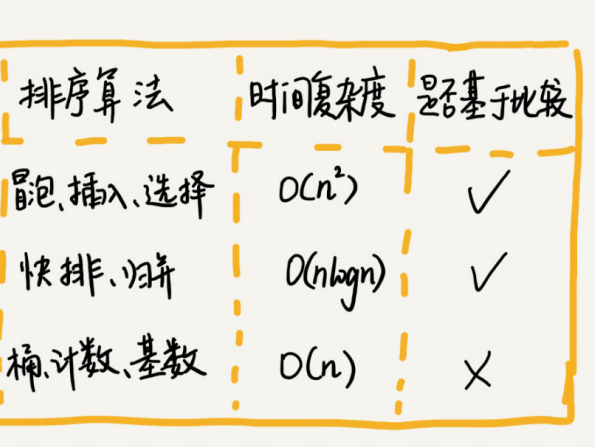## O(n2)级别的排序

### 冒泡排序（Bubble Sort）

public static void BubbleSort(int[] array){
int length = array.length;
//交换元素开始
for(int i=0; i<length; i++){
//每次排完一遍之后可以少做一次交换
boolean flag = false;
for(int j=0; j<length-i-1; j++){
if(array[j]>array[j+1]){
int temp = array[j];
array[j] = array[j+1];
array[j+1] = temp;
flag = true;
}
}
if (!flag)//当发现元素没有交换时，直接停止
break;
}
}


1. 冒泡的过程只涉及相邻数据的交换操作，只需要常量级的临时空间，所以它的空间复杂度为
O(1)，是一个原地排序算法
2. 在冒泡排序中，只有交换才可以改变两个元素的前后顺序。为了保证冒泡排序算法的稳定性，当
有相邻的两个元素大小相等的时候，我们不做交换，相同大小的数据在排序前后不会改变顺序，
所以冒泡排序是稳定的排序算法
3. 最好情况下，要排序的数据已经是有序的了，我们只需要进行一次冒泡操作，就可以结束了，所
以最好情况时间复杂度是 O(n)。而最坏的情况是，要排序的数据刚好是倒序排列的，我们需要
进行 n 次冒泡操作，所以最坏情况时间复杂度为 **O(n2)**平均也是。

### 插入排序

public static void insertSort(int[] arrays){
int n = arrays.length;
for(int i=1; i<n; i++){
int temp = arrays[i];
int j=i-1;
for(; j>=0; j--){
if(arrays[j]>temp){
arrays[j+1] = arrays[j];
}else {
break;
}
}
arrays[j+1] = temp;
}
}


1. 原地排序算法
2. 稳定的排序算法
3. 最好是时间复杂度为 O(n)。最坏情况时间复杂度为 O(n2)以平均时间复杂度为 O(n2)。

### 选择排序（Selection Sort）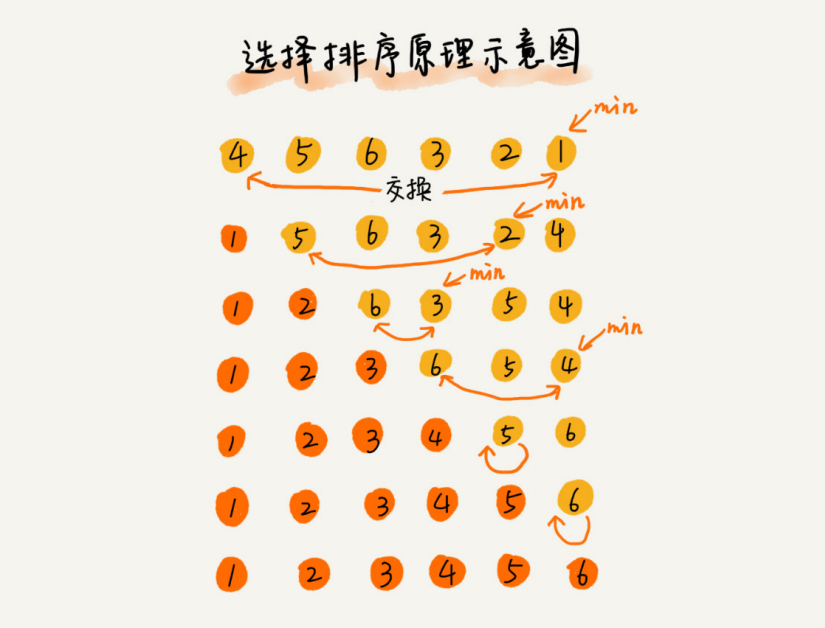public static void selectSort(int[] arr){
int temp = 0;
int flag = 0;
int n = arr.length;
for (int i = 0; i < n; i++) {
temp = arr[i];
for(int j=i+1; j<n; j++){
if(arr[j] < temp){
temp = arr[j];
flag = j;
}
}
arr[flag] = arr[i];
arr[i] = temp;
}
}


### 小结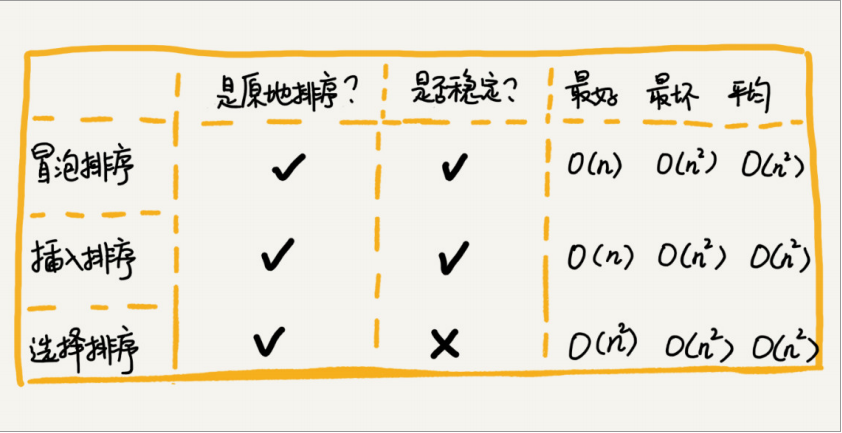## O(nlogn)级别的排序

### 归并排序（Merge Sort）

public static void mergeSort(int[] arr, int p, int r){
if(p<r){
int q = p+((r-p)>>1);
mergeSort(arr, p, q);
mergeSort(arr, q+1, r);
merge(arr, p, q, r);

}
}
public static void merge(int[] arr, int p, int q, int r){
int i,j,k,n1,n2;
n1 = q-p+1;
n2 = r-q;
int[] left = new int[n1];
int[] right = new int[n2];
//拷贝数组
for(i=0,k=p; i<n1; i++,k++)
left[i] = arr[k];
for (i=0,k=q+1; i<n2; i++,k++)
right[i] = arr[k];
//比较并拷贝到原数组中
for(k=p,i=0,j=0; i<n1 && j<n2; k++){
if(left[i]<=right[j]){
arr[k] = left[i];
i++;
}else {
arr[k] = right[j];
j++;
}
}
if(i<n1){
for(;i<n1;i++,k++)
arr[k] = left[i];
}
if(j<n2){
for(;j<n2;j++,k++)
arr[k] = right[j];
}
}


1. 归并排序是一个稳定的排序算法
2. 归并排序的时间复杂度任何情况下都是 O(nlogn)
3. 空间复杂度是 O(n)

### 快速排序算法（Quicksort）

public static void quickSort(int[] arr, int p, int r){
if (p >= r)
return;
int q = partition(arr, p, r);
quickSort(arr, p,q-1);
quickSort(arr,q+1,r);

}
public static int partition(int[] arr, int p, int r){
int index = arr[r];
int i = p;
int j = r;
while(i<j){
while(i<j && arr[i]<index){
i++;
}
if(i<j){
arr[j--] = arr[i];
}
while(i<j && arr[j]>index){
j--;
}
if(i<j){
arr[i++] = arr[j];
}
}
arr[i] = index;
return i;
}


1. 不稳定排序
2. 原地排序
3. 大部分情况下的时间复杂度都可以做到 O(nlogn)，只有在极端情况
下，才会退化到 O(n2)

### 番外篇

O(n) 时间复杂度内求无序数组中的第 K 大元素。比如，4， 2， 5， 12， 3 这样一组数据，第 3 大元素就是
4。

## O(n) 级别的排序算法

### 桶排序（Bucket sort）

10GB 订单数据是无法均匀地被划分到 100 个文件中的。有可能某个金额区间的数据特别多，

### 计数排序（Counting sort）

C 内存储的并不是考生，而是对应的考生个数。像我刚刚举的那个例子，我们只需要遍历一7，也就是说，到目前为止，包括自己在内，分数小于等于 3 的考生有 7 个，也就是说 3 是数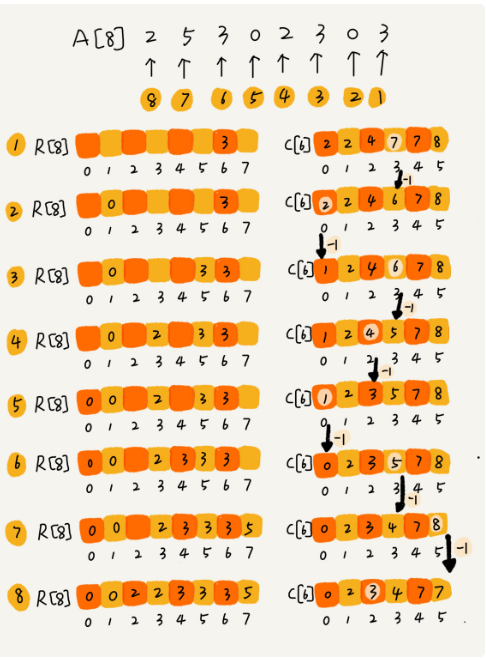## 如何实现一个通用的、高性能的排序函数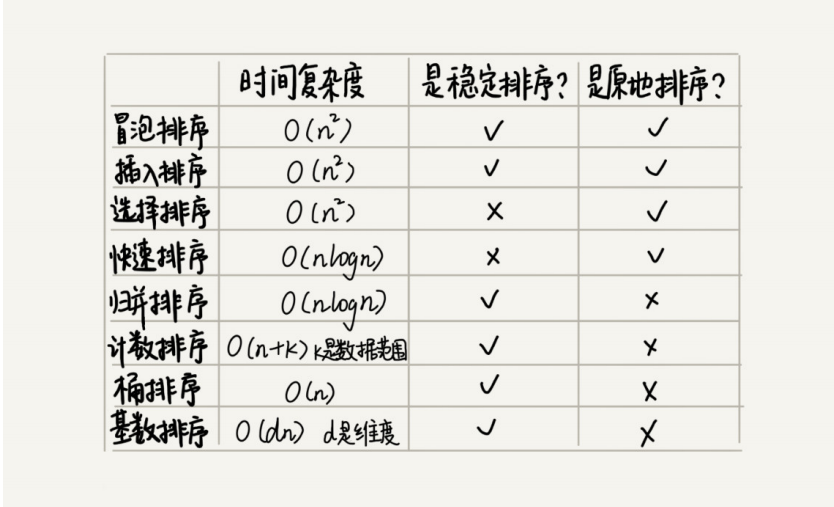©️2019 CSDN 皮肤主题: 大白 设计师: CSDN官方博客